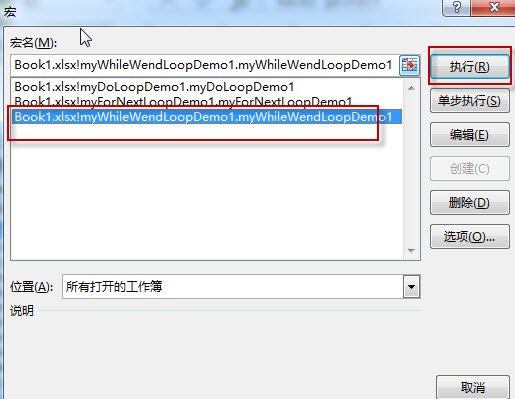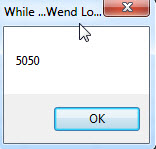## Excel VBA Loops

This post will guide you how to use loops statements in MS excel VBA. There are three most useful Loops statements as bellows: For …Next loop, Do … Loop and While …Wend loop.

You can use Loop statement to perform the same statement multiple times in MS VBA program.

If we want to add up the numbers 1 to 10, we can easily to get the results. But if we need to add up the numbers 1 to a 100. You wouldn’t have to type them all out to do addition operation, now we can use the loop.

### For … Next Loop example

Sample1: add up the numbers 1 to 100

1# open visual Basic Editor, then insert a module and name as: myForNextLoopDemo1

2# enter into the below VBA codes in code window.

```Sub myForNextLoopDemo1()
Dim i As Integer, iSum As Integer
iSum = 0
For i = 1 To 100
iSum = iSum + i
Next
MsgBox iSum, , "For ...Next Loop"
End Sub```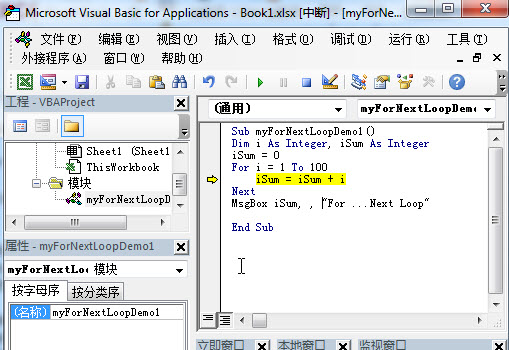3# back to workbook and run the above macro.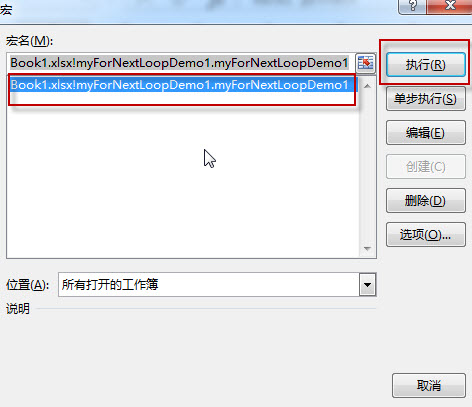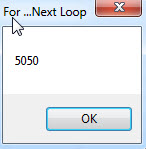### Do … Loop example

Sample2: add up the numbers 1 to 100 using Do Loop

1# open visual Basic Editor, then insert a module and name as: myDoLoopDemo1

2# enter into the below VBA codes in code window.

```Sub myDoLoopDemo1()

Dim i As Integer, iSum As Integer

iSum = 0

i = 1

Do Until i > 100

iSum = iSum + i

i = i + 1

Loop

MsgBox iSum, , "Do ...Loop"

End Sub```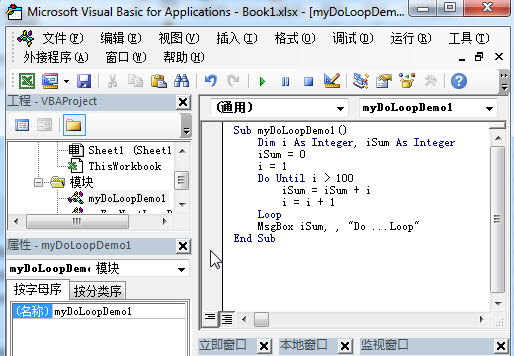3# back to workbook and run the above macro.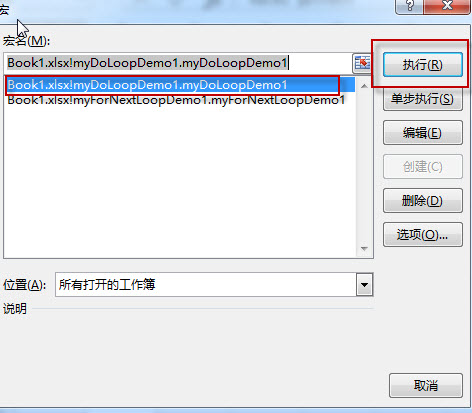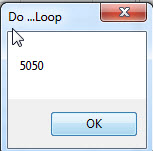### While …Wend loop Example

Sample3: add up the numbers 1 to 100 using While … Wend Loop

1# open visual Basic Editor, then insert a module and name as: myWhileWendLoopDemo1

2# enter into the below VBA codes in code window.

```Sub myWhileWendLoopDemo1()

Dim i As Integer, iSum As Integer
iSum = 0
i = 1
While i < 101
iSum = iSum + i
i = i + 1
Wend
MsgBox iSum, , "While ...Wend Loop"
End Sub```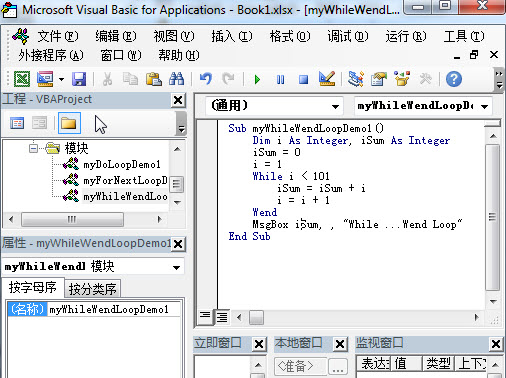3# back to workbook and run the above macro.